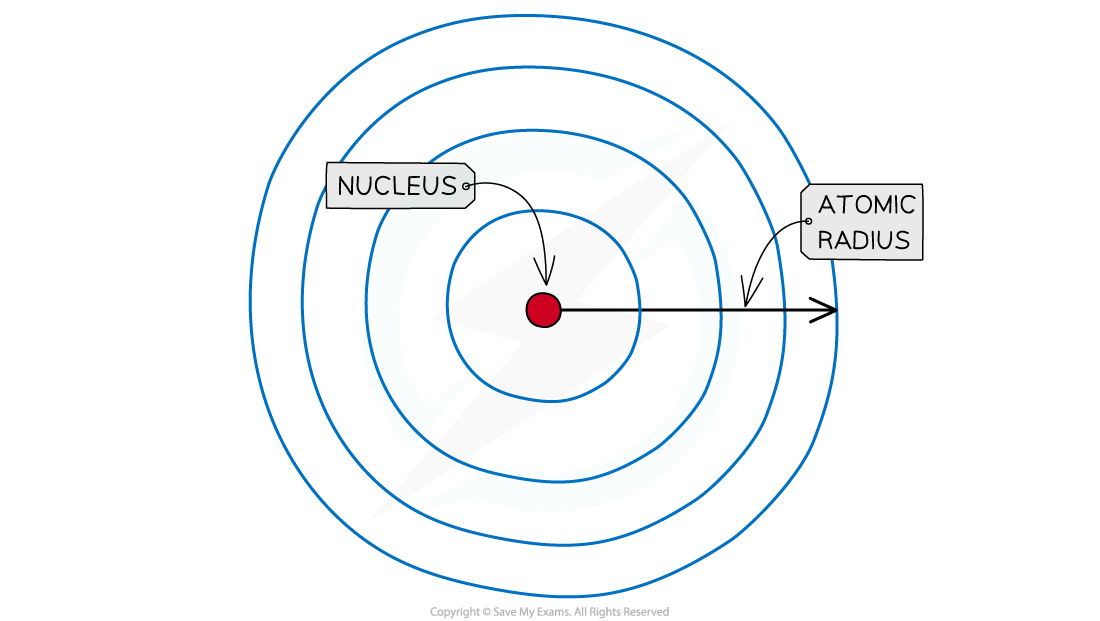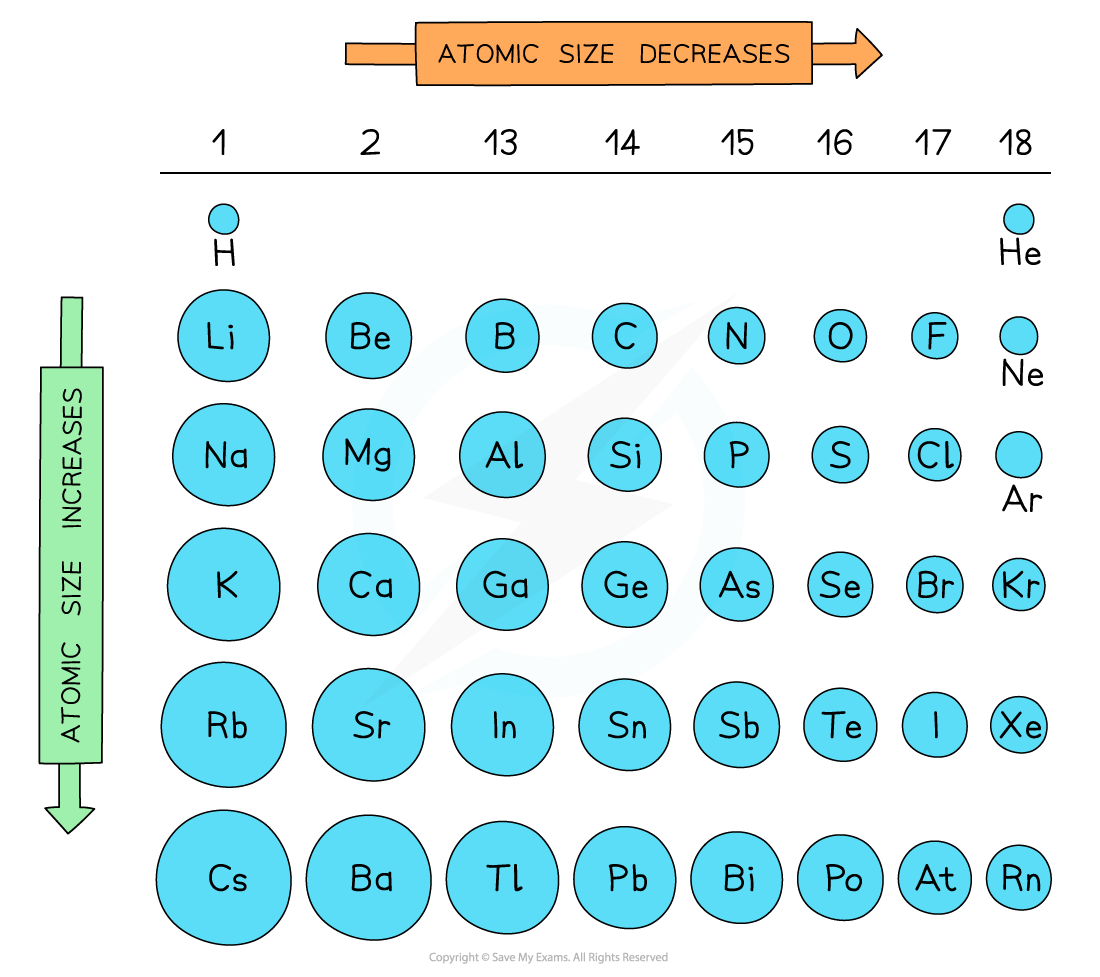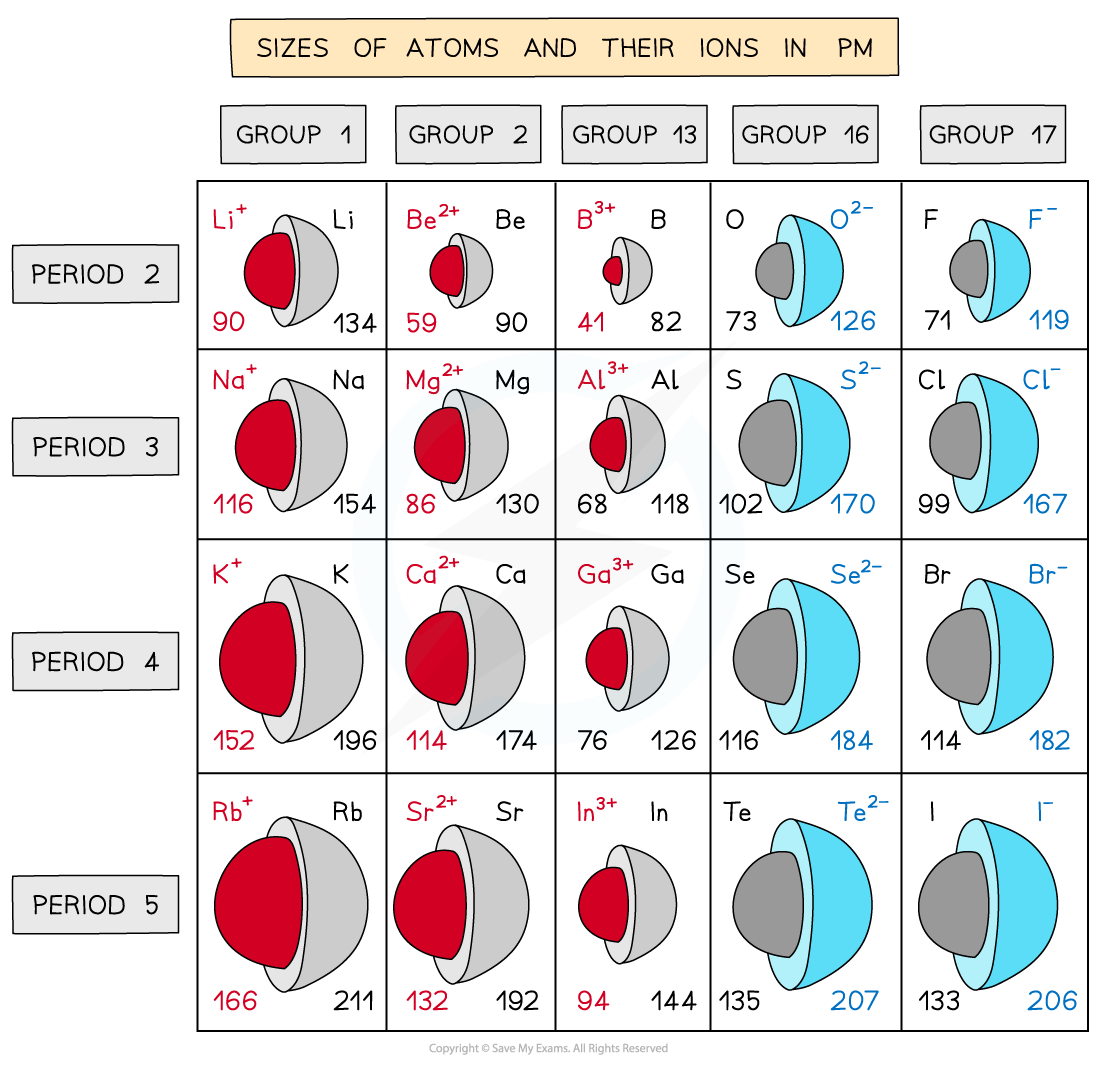# IB DP Chemistry: SL复习笔记3.1.2 Periodic Trends: Physical - Atomic & Ionic Radius

• The atomic radius of an element is a measure of the size of an atom
• It is the distance between the nucleus of an atom and the outermost electron shell
• It can be quite hard to determine exactly where the boundary of an atom lies, so a variety of approches are taken such as half the mean distance between two adjacent atoms
• This will vary depending on the type of structure and bonding, but it gives a comparative value for atomsThe atomic radius of an atom is the typical distance between the nucleus and the outermost electron shell

• Atomic radii show predictable patterns across the periodic table
• They generally decrease across each period
• They generally increase down each group
• These trends can be explained by the electron shell theory
• Atomic radii decrease as you move across a period as the atomic number increases (increased positive nuclear charge) but at the same time extra electrons are added to the same principal quantum shell
• The larger the nuclear charge, the greater the pull of the nuclei on the electrons which results in smaller atoms
• Atomic radii increase moving down a group as there is an increased number of shells going down the group
• The electrons in the inner shells repel the electrons in the outermost shellsshielding them from the positive nuclear charge
• This weakens the pull of the nuclei on the electrons resulting in larger atomsTrends in the atomic radii across a period and down a group

• The diagram shows that the atomic radius increases sharply between the noble gas at the end of each period and the alkali metal at the beginning of the next period
• This is because the alkali metals at the beginning of the next period have one extra principal quantum shell
• This increases shielding of the outermost electrons and therefore increases the atomic radius

• The ionic radius of an element is a measure of the size of an ion
• Ionic radii show predictable patterns
• The trend down a group is the same as atomic radius - it increases as the number of shells increases
• The trend across a period is not so straightforward as it depends on whether it is positive or negative ions being considered
• Ionic radii increase with increasing negative charge
• Ionic radii decrease with increasing positive charge
• These trends can also be explained by the electron shell theory
• Ions with negative charges are formed by atoms accepting extra electrons while the nuclear charge remains the same
• The extra electrons experience repulsion with the other valence electrons which increases the ionic radius
• The greater the negative charge, the larger the ionic radius
• Positively charged ions are formed by atoms losing electrons
• The nuclear charge remains the same but there are now fewer electrons which undergo a greater electrostatic force of attraction towards the nucleus which decreases the ionic radius
• The greater the positive charge, the smaller the ionic radiusTrends in the ionic radii across a period and down a group

#### Worked Example

Which option shows atoms in order of decreasing atomic radius?

A. N > C > Be > Mg

B. Mg > N > C > Be

C. Be > C > N > Mg

D. Mg > Be > C > N

Option D is the correct answer

• First, you need to identify that Be, C and N are all in period 2, but Mg is in period 3, so Mg will have the biggest radius.
• Secondly, atomic radius decreases across the period so Be, C and N decrease in that order as they belong to groups 2, 14 and 15, respectively